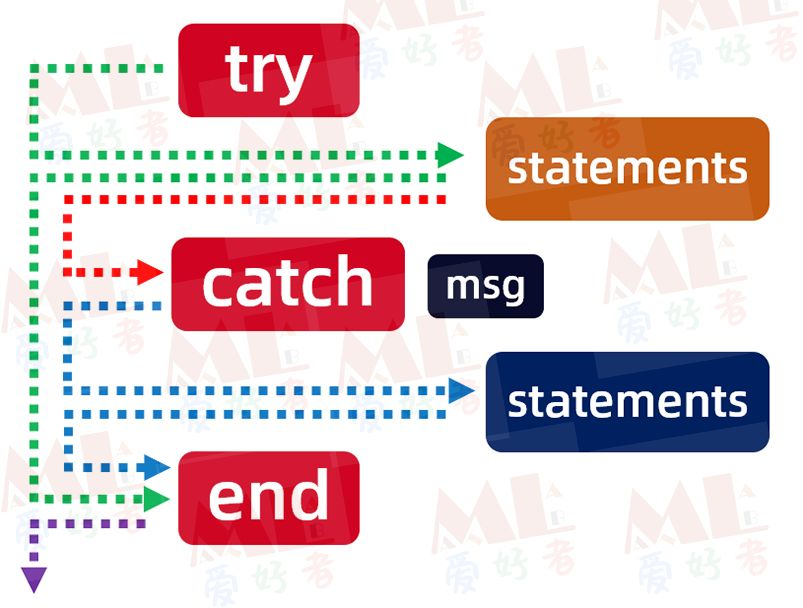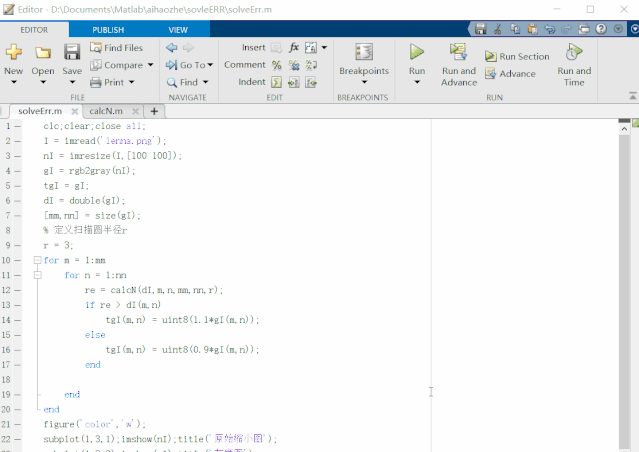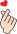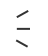# 如何在matlab编程中逢山开路、遇水搭桥？Error! Error! Error!clc;clear;close all;
% 修改图像尺寸
nI = imresize(I,[100 100]);
% 转换成灰度图
gI = rgb2gray(nI);
tgI = gI;
% 强制类型转换以便后续计算
dI = double(gI);
[mm,nn] = size(gI);
% 定义扫描圆半径r
r = 3;
for m = 1:mm
for n = 1:nn
re = calcN(dI,m,n,mm,nn,r);
if re > dI(m,n)
tgI(m,n) = uint8(1.1*gI(m,n));
else
tgI(m,n) = uint8(0.9*gI(m,n));
end

end
end
figure('color','w');
subplot(1,3,1);imshow(nI);title('原始缩小图');
subplot(1,3,2);imshow(gI);title('灰度图');
subplot(1,3,3);imshow(tgI);title('处理图');


calcN函数：

function re = calcN(I,M,N,mm,nn,R)
re = 0;
k = 0;
for m = M-R:M+R
for n = N-R:N+R
try
% 索引值小于等于0，或者越界时，程序都将出错
% 这里使用try...catch...end巧妙避开了错误索引，
% 而只计算矩阵大小范围内索引值，比使用if语句更简洁
tmp = I(m,n);
tR = norm([M,N]-[m,n]);
if tR < R && m ~= mm && n ~= nn
k = k + 1;
re = re+tmp;
end
catch
disp('当前索引值越界');
end
end
end
re = re/k;https://ww2.mathworks.cn/help/matlab/ref/try.html

### 往期回顾>>>>>>©️2019 CSDN 皮肤主题: 编程工作室 设计师: CSDN官方博客